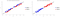# Limitations, Assumptions Watch-Outs of Principal Component Analysis

Hey!, I know enough of PCA, but why not?

It is a great algorithm with certain watch-outs which can mostly be tackled with certain adjustments in the Vanilla PCA.

# ENJOY!!

• Check whether some assumptions hold, like the presence of linear correlations (e.g., Bartlett’s test of sphericity) or sampling adequacy (e.g., Kaiser-Meyer-Olkin test). The distinctiveness of the eigenvalues is a fundamental assumption of PCA so that Principal Components are unique. The following image was taken from here:
• Τhe method relies on linear relationships between the variables in a dataset. So, what if there are correlations but are not linear? There is the so-called called kernel PCA version that allows PCA to also work with non-linear data. Vanilla PCA computes the covariance matrix of the dataset:

Kernel PCA, on the other hand, first transforms the data into an even higher-dimensional space where: C

And only then projects the data onto the eigenvectors of that matrix, just like regular PCA. The kernel trick refers to performing the computation without actually computing Φ(x). This is possible only if Φ is chosen such that it has a known corresponding kernel. KPCA doesn’t always cut it, so depending on your dataset, you may need to look at other non-linear dimensionality reduction techniques, such as LLE, isomap, or t-SNE.

• Assumption of orthogonality, since the principal components are by design orthogonal to each other. But far “better” basis vectors may exist to summarize the data that are not orthogonal. The following image shows an extreme such case that was taken from here:
• Large variance is used as a criterion for the for search of PCs. However, sometimes structure is found in places with low variance, as we see in the following image. If we kept only the first principal component, we would be absolutely fine in the right case, but in the left case, we would perform badly in a classification context.PCA fails in Left figure
• PCA is scale variant. This means that if the variables in our dataset have different units, some variables will dominate the others simply because they assume bigger values, and therefore contribute more to the overall variance. That’s why we typically transform our data so that they have a unit standard deviation. However, this may or may not be appropriate depending on the research question. E.g., if we are doing PCA on gene expression data, this would put an equal “weight” on each gene. Again, this may or may not be desired. (Also, the data absolutely need to be mean-centered)
• The point of PCA is to reduce the dimensionality of a dataset. So, how do we decide how many principal components to retain? Approaches often used include visual inspection of the scree plot looking for an “elbow”, keeping components accounting for a fixed amount of the total variance, e.g., 95% of the total variance, or picking components with eigenvalues > 1.One technique is to use the “broken stick” model. The idea behind this is to model N variances by assuming a stick of unit length and breaking it into N pieces by randomly (and simultaneously) selecting break points from a uniform distribution. We then compare element-wise the percentage variances of our components against the percentages from the broken stick distribution. As long as observed eigenvalues are higher than the corresponding random broken stick components, we keep the principal components. See for example the following figure where the broken stick model is compared with the eigenvalue > 1 criterion. Another method is to use the bootstrap resampling technique to calculate confidence intervals for the eigenvalues and keep those whose CI contains 1.
• One very important issue is that of interpretability. Once we have replaced our original variables with the principal components, it’s not always entirely trivial to interpret the results.
1. Sometimes, depending on the data’s structure and the research question, one might apply a rotation after PCA to simplify the components’ interpretation. Such rotations include Varimax and oblique rotations (however, these have their own set of limitations, e.g., they might produce components that don’t correspond to successive maximal variance or produce components that are correlated or give slightly different results every time they are applied given that they are iterative methods).
2. Another path to simplifying PCs, therefore to interpretation, is to force additional constraints on the new variables, e.g., a direct L1 constraint. Or reformulate PCA as a regression problem and use LASSO. Either way, that’s the field of Sparse PCA.

# MY SOCIAL SPACE

Problem Solver, Data Science, Actuarial Science, Knowledge Sharer, Hardcore Googler

## More from Shaily jain

Problem Solver, Data Science, Actuarial Science, Knowledge Sharer, Hardcore Googler